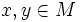# Homogeneous metric

This article defines a property that makes sense for a Riemannian metric over a differential manifold

## Definition

### Given data

A differential manifold$M$ equipped with a Riemannian metric$g$.

### Definition part

The metric$g$ is said to be a homogeneous metric if given any points$x,y \in M$, there exists an isometry of$M$ sending$x$ to$y$.